# Question & Answer: Create a web page that allows a user to enter as many numbers as desired. The application will separate the input into even and odd numbers……

Create a web page that allows a user to enter as many numbers as desired. The application will separate the input into even and odd numbers.

1. HTML Dynamic Content – Output (2 marks) The application will display the sum and average of the even numbers.

Don't use plagiarized sources. Get Your Custom Essay on
Question & Answer: Create a web page that allows a user to enter as many numbers as desired. The application will separate the input into even and odd numbers……
GET AN ESSAY WRITTEN FOR YOU FROM AS LOW AS \$13/PAGE

2. HTML Dynamic Content – Output (2 marks) The application will display the sum and average of the odd numbers.

3. HTML Static Content – Instructions (1 marks) Be sure to explain to the user what your web application is supposed to do. Include details on how to use the application.

4. CSS (1 mark) Apply CSS to improve the user experience. Ensure that the output is nicely formatted.

5. JavaScript – Data Validation (1 mark) Do not accept an input of ’0’.

6. JavaScript – Data Validation (1 mark) Do not accept non-numerical user input.

7. Programming Style and Standards (1 mark) It is a good idea to practice conforming to a set of programming standards. Refer to the posted summary of the Programming Standards used by the CP/CPA programs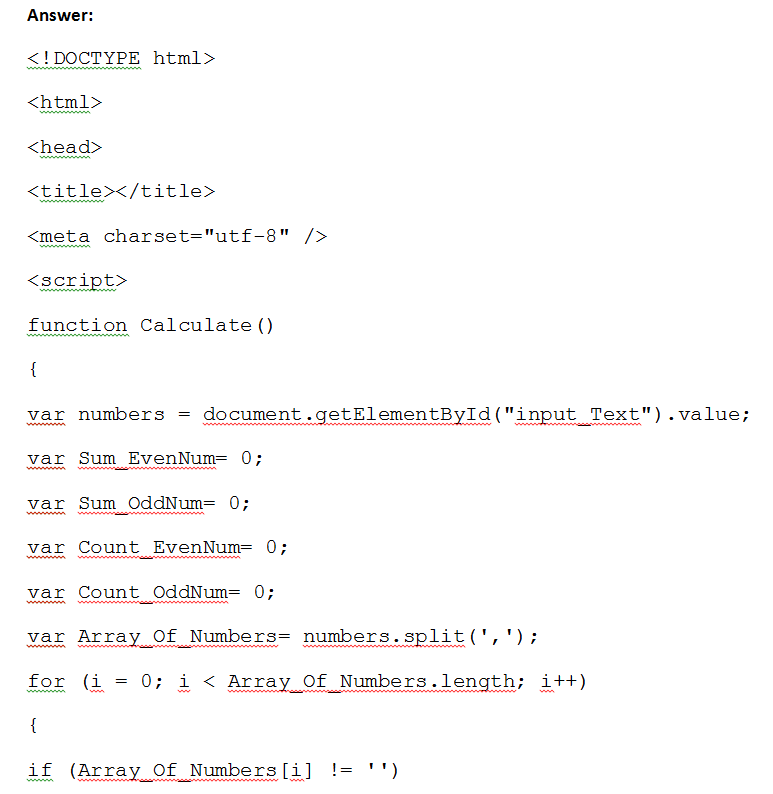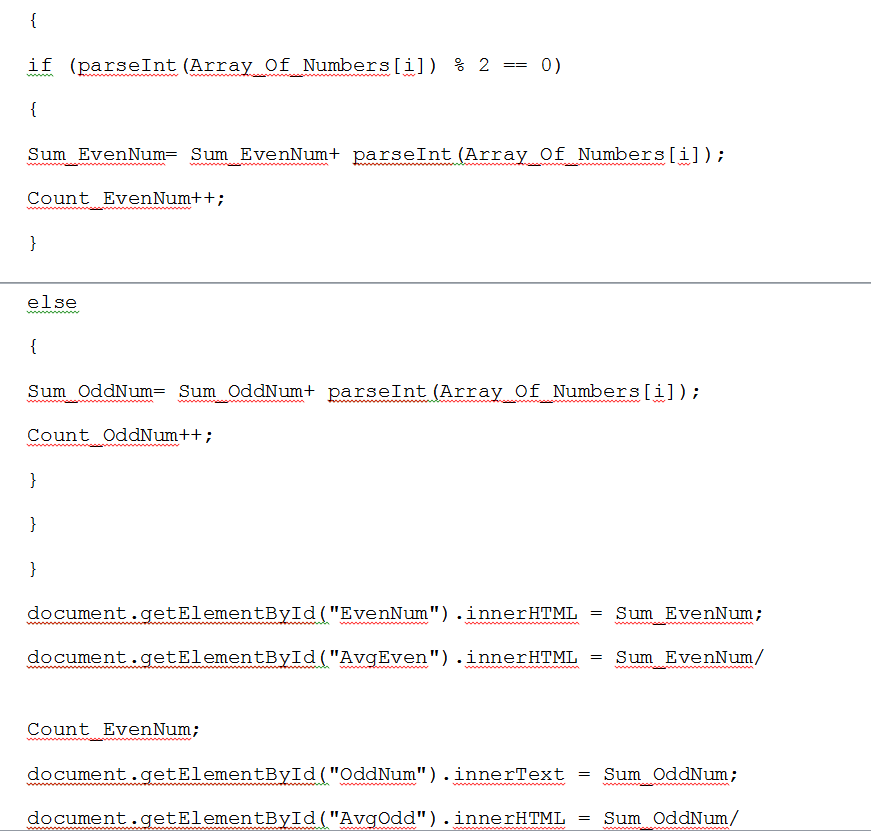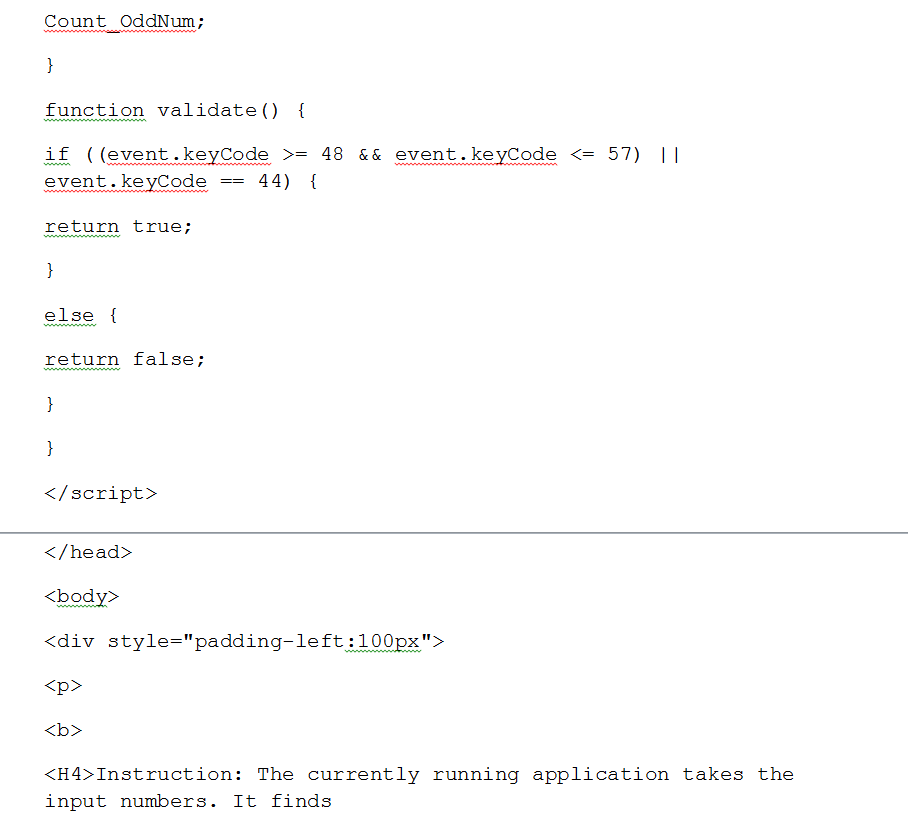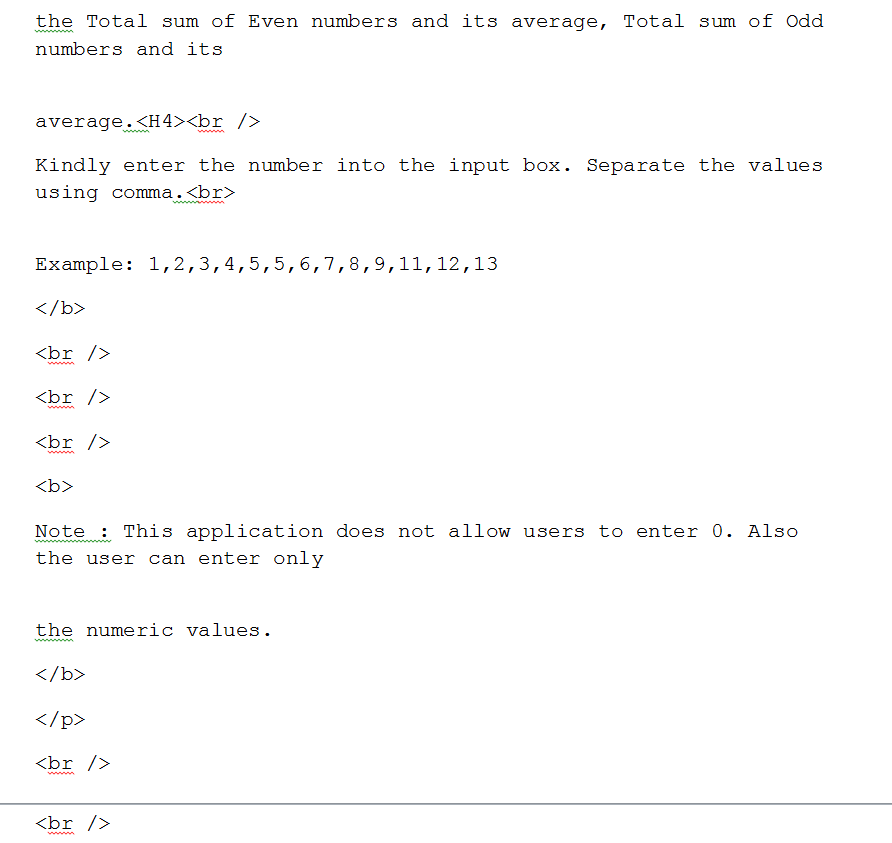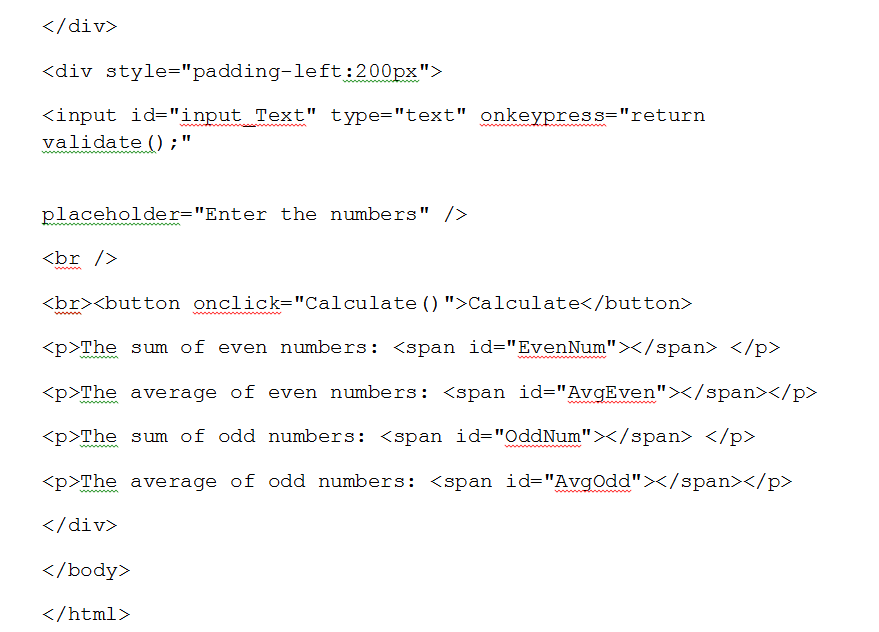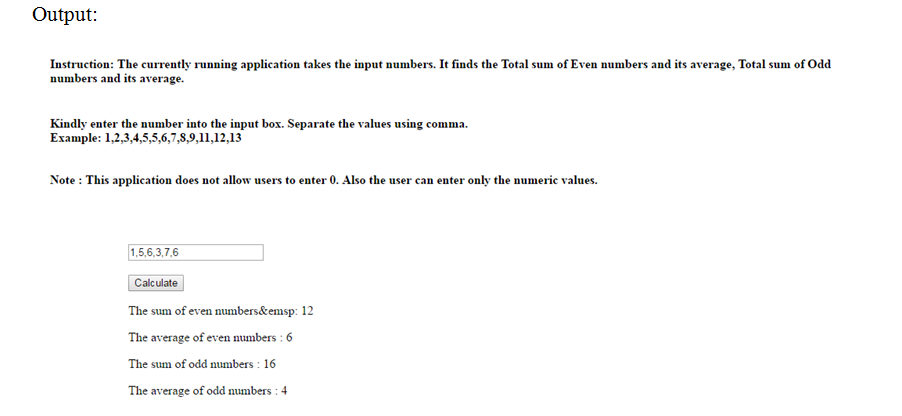Note: paste the below code in the notepad and save it as “app.html”. Open the html file with any browser. Here I opened with chrome.

Editable Code:

<!DOCTYPE html>
<html>
<title></title>
<meta charset=”utf-8″ />
<script>
function Calculate()
{
var numbers = document.getElementById(“input_Text”).value;
var Sum_EvenNum= 0;
var Sum_OddNum= 0;
var Count_EvenNum= 0;
var Count_OddNum= 0;
var Array_Of_Numbers= numbers.split(‘,’);
for (i = 0; i < Array_Of_Numbers.length; i++)
{
if (Array_Of_Numbers[i] != ”)
{
if (parseInt(Array_Of_Numbers[i]) % 2 == 0)
{
Sum_EvenNum= Sum_EvenNum+ parseInt(Array_Of_Numbers[i]);
Count_EvenNum++;
}
else
{
Sum_OddNum= Sum_OddNum+ parseInt(Array_Of_Numbers[i]);
Count_OddNum++;
}
}
}
document.getElementById(“EvenNum”).innerHTML = Sum_EvenNum;
document.getElementById(“AvgEven”).innerHTML = Sum_EvenNum/

Count_EvenNum;
document.getElementById(“OddNum”).innerText = Sum_OddNum;
document.getElementById(“AvgOdd”).innerHTML = Sum_OddNum/

Count_OddNum;
}
function validate() {
if ((event.keyCode >= 48 && event.keyCode <= 57) || event.keyCode == 44) {
return true;
}
else {
return false;
}
}
</script>
<body>
<p>
<b>
<H4>Instruction: The currently running application takes the input numbers. It finds

the Total sum of Even numbers and its average, Total sum of Odd numbers and its

average.<H4><br />
Kindly enter the number into the input box. Separate the values using comma.<br>

Example: 1,2,3,4,5,5,6,7,8,9,11,12,13
</b>
<br />
<br />
<br />
<b>
Note : This application does not allow users to enter 0. Also the user can enter only

the numeric values.
</b>
</p>
<br />
<br />
</div>